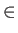Next: Evasive Functions: Up: Preliminaries Previous: Preliminaries   Contents

#### Deterministic Decision Trees:

A decision tree of N bits is a rooted binary tree. Each internal node of the tree contains an index i{1,..., N} and has two children. Each leaf contains either a 1 or a 0. To determine the value of the deterministic decision tree on an input x{0, 1}N we traverse the tree starting at the root. At each internal node we examine the xi, where i is the index stored at that node. If xi = 1 we follow the path through the left subtree; if xi = 0 we follow the path through the right subtree. The value stored at the leaf that we eventually reach is the output of the algorithm. Any decision tree computes an N-bit Boolean function. There are many different deterministic decision trees that compute any given function.

The decision tree complexity of a Boolean function is the minimum depth of any decision tree that computes that function. We denote the decision tree complexity of a Boolean function f by D(f ). The decision tree complexity of a function f is just its classical oracle query complexity. Any decision tree of depth D(f ) determines a minimal sequence of oracle queries that allow us to compute f (x) for any input x.Next: Evasive Functions: Up: Preliminaries Previous: Preliminaries   Contents
Matthew Hayward Lower Query Bounds in the Quantum Oracle Model GitHub Repository